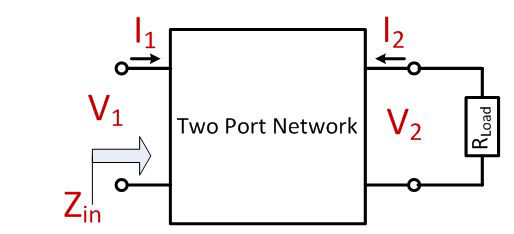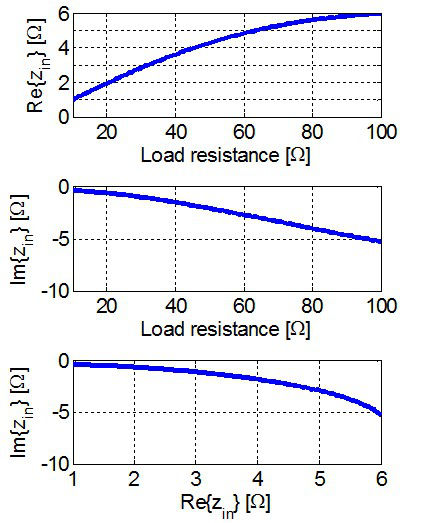LIBRARY

# Discussion on the Compression of the Resistance Range in Two-port Networks

Year: 2014Fig. 1. LTI lossless two port network.
Load variations are a known problem for resonant conversion systems. With a wide range of variations, each requires a significant change in the switching frequency, complicating EMI compatibility design, and efficiency that would reduce especially in light load conditions. The load variation problem can be significantly reduced with use of dedicated circuitry that would "compress" the variation of the load resistance into a smaller range. Current effort in the scientific community examines a structure that utilizes a passive, lossless network with the combination of passive rectifiers. Although the structure (called resistance compression network) is simple and significant suppression of the load variations is possible, the structure is only applicable to DC-DC conversion systems, where the same effect could be achieved if the active solution had been pursued instead.

Thus, this work examines the existence and practicality of the resistance compression networks for resonant power conversion. The investigation has been carried out generalizing a compression network as the Linear, Time-Invariant, lossless two-port network (Fig. 1). ABCD parameters have been used in the analysis. After some mathematical derivations, it was found that such a range in a compression circuit cannot be synthetized. Fig. 2 summarizes the issue. Although the compression can be achieved when looking at the real part of the input impedance (range [10 ω, 100 ω] compressed to [1 ω, 6 ω]), it is not possible to keep the imaginary part of the impedance small. The solution has to be found as a time-variant circuit and one such practical topology will be presented in the full paper, namely a resistive point-compressor, where a range of resistance is compressed to a single point only.Fig. 2. Parasitic inductance with different switching loops.